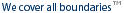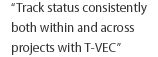# Test Design

## Function (Model-based) versus Structural

Testing strategies are typically characterized as either structural or functional. Structural testing relates directly to an implementation. Functional testing is based on a specification or model. Model-based testing relies on properties of the software defined by the model, as opposed to the source code. Model-based approaches extend implementation-based testing techniques to requirement models. The level of confidence that the program actually satisfies the model is based on the completeness and correctness of the derived tests with respect to the model, and the completeness and consistency between the model and the implementation.

## Test Selection

T-VEC utilizes a functional strategy based on requirements and design models. T-VEC's tools form a model-based test generation and model-analysis system. Its mechanisms are related to the most widely accepted testing concepts and strategies, based on classifications of errors that commonly result in program faults. These are either "computation" errors or "domain" errors. A computation error occurs when the correct path through the program is executed but the output is incorrect due to computation faults in the code. A domain error occurs when the logic path through the program is incorrect. In other words, a domain error occurs when the program makes the wrong decision. A computation error occurs when the program does the math incorrectly. These terms were defined by by Zeil and Howden.

## Domain Testing

White and Cohen proposed domain testing theory as a strategy for selecting test points to reveal domain, or decision-type, errors. T-VEC's test selection strategies are based on this theory, which holds that there is a strong correlation between constraints in the model and decisions in the program. The theory is based on the premise that if there is no coincidental correctness, then test cases that localize the boundaries of domains with arbitrarily high precision are sufficient to test all the points in the domain. T-VEC test selection heuristics enhance the domain testing principles to support detecting computation errors. Specifications are transformed into a set of a AND/OR logic structures known as disjunction normal form (DNF). Each DNF partition represents a unique requirement that must be tested. Test points are selected on the domain boundaries associated with each DNF partition. The resulting tests exercise the extreme boundaries for each decision along a program control path. In addition, they stress the max and minimum values of the unconstrained variables to detect computation errors.